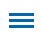First graders begin to work with addition and subtraction equations. Although these equations mostly involve one-digit numbers, the opportunity to add and subtract multiple of ten (with numbers less than 100) is available. The Equation Board Help template assesses student knowledge of the skill.

Editor's Note: This blog is part of a series of posts from former math specialist Scott Loomis on the real world math templates he has created for Wixie and how you can use and modify them for your own awesome performance tasks. You can read the backstory on his experience and expertise below.

The Equation Board Help template asks students to 'correct' three different display boards that involve addition and subtraction equations as true or false.Wixie Equation Board Help Template

As always, Wixie makes it easy to open and customize existing templates. We encourage you to customize the template to modify the existing problems and activities to meet your specific computation expectations.

Instructional Concept: Work with addition and subtraction equations

Template Location in Wixie: Curriculum > Math > Algebra

Estimated Completion Time: 10-20 minutes

# Math Standards and Learning Goals - Grade 1

Common Core State Standards - Mathematics - Operations & Algebraic Thinking
1.OA.D.7 Understand the meaning of the equal sign and determine if equations involving addition and subtraction are true or false. For example, which of the following equations are true and which are false? 6 = 6, 7 = 8 - 1, 5 + 2 = 2 + 5, 4 + 1 = 5 + 2.

Virginia SOL - Mathematics - Patterns, Functions, and Algebra
1.18 The student will demonstrate an understanding of equality through the use of the equal sign.

Texas TEKS - Mathematics -Algebraic Reasoning
The student applies mathematical process standards to identify and apply number patterns within properties of numbers and operations in order to describe relationships. The student is expected to:
(E) understand that the equal sign represents a relationship where expressions on each side of the equal sign represent the same value(s)

## Wixie Skills Review:

Students: The completion of this assessment is fairly straightforward as students simply click and drag objects. On the third page, students must modify an existing text box before (or after) moving it to the desired location. This can be done by simply double-clicking in each blue text object.

Teachers: It is important for teachers to utilize this template to best meet the mathematical understanding of their students. For some, this may involve modifying existing equations to add and subtract multiple of 10 when using numbers below 100. For others, it may involve simplifying the process by removing some of the problems. However, modifying the existing equations can be tricky as the objects have been both Un-Scaled and Protected to limit the amount of unwanted modification by students.

For the first page of the Equation Board Help template, students are asked to move the True/False statement to correctly represent the given equations. The equations have been Glued to eliminate unwanted student movement. Modification of these involves painting (maybe a white rectangle?) over each equation and inserting a new text box.

The second page involves a similar process as equations, and True/False statements within the board have been Glued. However, the text objects 'below' the board have been Protected and Un-Scaled. To modify these, select the desired text object and from the Edit menu, choose Properties and unselect Protect. If you wish to modify the shape of the text object then also select Scale.

## Making your own custom templates in Wixie

Wixie is a powerful canvas for elementary math learning. Wixie makes it easy to combine typed and drawn numbers, with math manipulatives and audio explanations of mathematical thinking.

With limited classroom time, you can create or customize Wixie templates to guide student math practice and independent work to meet specific math goals in your classroom.

Topics: Wixie, real world of math